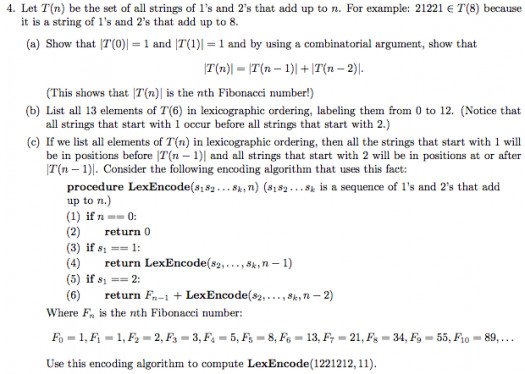# Do You Know Combinatorial Number?

Approved & Edited by ProProfs Editorial Team
The editorial team at ProProfs Quizzes consists of a select group of subject experts, trivia writers, and quiz masters who have authored over 10,000 quizzes taken by more than 100 million users. This team includes our in-house seasoned quiz moderators and subject matter experts. Our editorial experts, spread across the world, are rigorously trained using our comprehensive guidelines to ensure that you receive the highest quality quizzes.
| By Livyn
L
Livyn
Community Contributor
Quizzes Created: 347 | Total Attempts: 144,443
Questions: 10 | Attempts: 41SettingsIn mathematics, and in particular in combinatorics, the combinatorial number system of degree k (for some positive integer k), also referred to as combinadics, is a correspondence between natural numbers (taken to include 0) N and k-combinations, represented as strictly decreasing sequences ck > . . . > c2 > c1 ≥ 0.

• 1.

### Combinatorial number is studied in ......?

• A.

Mathematics

• B.

Language

• C.

Music

• D.

History

A. Mathematics
Explanation
Combinatorial numbers are studied in mathematics. Combinatorics is a branch of mathematics that deals with counting, arranging, and selecting objects. Combinatorial numbers, also known as binomial coefficients, are used to count the number of ways to choose a subset of objects from a larger set. They have applications in various fields such as probability, algebra, and computer science. Therefore, the correct answer is mathematics.

Rate this question:

• 2.

### The originally used term "combinatorial representation of integers" is shortened to "combinatorial number system" by?

• A.

Knuth

• B.

D.H Lehmer

• C.

James McCaffrey

• D.

Isaac Newton

A. Knuth
Explanation
The correct answer is Knuth. Knuth, a computer scientist and mathematician, is known for his extensive work in the field of combinatorial algorithms. He is credited with popularizing the term "combinatorial number system," which is a shortened version of the original term "combinatorial representation of integers." Knuth's contributions to combinatorial mathematics have had a significant impact on the field and his name is often associated with this concept.

Rate this question:

• 3.

### Distinct numbers correspond to distinct k-combinations, and produce them in .......?

• A.

Lexicographic order

• B.

Alphabetic order

• C.

Chronological order

• D.

Numeric order

A. Lexicographic order
Explanation
Distinct numbers correspond to distinct k-combinations, meaning that each combination is unique and does not repeat. Lexicographic order is a way of arranging these combinations in a specific order, where the elements are ordered based on their position in the dictionary. This order is determined by comparing the elements from left to right, and arranging them based on their respective positions in the alphabet or numerical value. Therefore, the correct answer is lexicographic order.

Rate this question:

• 4.

### The fact that a unique sequence so corresponds to any number N was observed by?

• A.

James McCaffrey

• B.

Isaac Newton

• C.

Knuth

• D.

D.H Lehmer

D. D.H Lehmer
Explanation
D.H Lehmer observed that a unique sequence corresponds to any number N.

Rate this question:

• 5.

### The term "combinadic" is introduced by who?

• A.

James McCaffrey

• B.

Isaac Newton

• C.

Knuth

• D.

D.H Lehmer

A. James McCaffrey
Explanation
James McCaffrey is the one who introduced the term "combinadic".

Rate this question:

• 6.

### The main purpose of the combinatorial number system is to provide a/an ......., each by a single number, of all {\displaystyle {\tbinom {n}{k}}} possible k-combinations of a set S of n elements

• A.

Representation

• B.

Imitation

• C.

Application

• D.

Emuneration

A. Representation
Explanation
The main purpose of the combinatorial number system is to provide a representation, each by a single number, of all possible k-combinations of a set S of n elements.

Rate this question:

• 7.

### One could use the combinatorial number system to list or traverse all k-combinations of a given ........?

• A.

Characteristic vector

• B.

Infinite set

• C.

Finite set

• D.

Assembly code

C. Finite set
Explanation
The combinatorial number system can be used to list or traverse all k-combinations of a given finite set. This system assigns a unique number to each combination, allowing for an efficient way to generate and iterate through all possible combinations. In contrast, an infinite set cannot be exhaustively listed or traversed using this system, as it would require an infinite number of combinations. Similarly, assembly code and characteristic vectors are not directly related to the combinatorial number system.

Rate this question:

• 8.

### Corresponding assembly code was described as item 175 in .....?

• A.

Characteristic vectors

• B.

HAKMEM

• C.

• D.

Primorial number system

B. HAKMEM
Explanation
The corresponding assembly code was described as item 175 in the HAKMEM.

Rate this question:

• 9.

### Corresponding assembly code was described as ....... in HAKMEM.

• A.

Item 160

• B.

Item 175

• C.

Item 145

• D.

Item 188

B. Item 175
Explanation
In the HAKMEM document, the corresponding assembly code was described as "item 175".

Rate this question:

• 10.

### Factorial number system is also known as?

• A.

• B.

Primorial number system

• C.

Combinatorial number system

• D.

BijectionBack to top